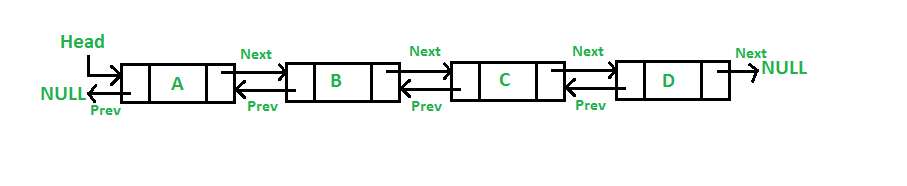Related Tags

python
communitycreator
data structures

### Overview

A LinkedList is a collection of nodes that together represent a sequence.

• It is a dynamic and linear data structure. It doesn’t need a contiguous memory location.

• It can be visualized as a chain of nodes where each node contains a data field and a reference to the next node in the list.

• LinkedList can be further classified into:

1. Singly LinkedList
2. Doubly LinkedList

### What is Traversal()?

Traversal() is used to visit each node of the list to perform an operation. Here, we will traverse and print data present in the list.

### Singly LinkedList

A singly LinkedList is Uni-directional, meaning traversal is possible in forwarding direction only.### Example

#Traversing using singly linked list
class Node:                   #Creating a node
def __init__(self, data=None):
self.data = data
self.next = None

def __init__(self):

def Traversal(self):   #Traversal through linked List
while n is not None:
print (n.data)
n = n.next

l2 = Node("Tuesday")
l3 = Node("Wedneday")

# Link first Node to second node
# Link second Node to third node
l2.next = l3
list.Traversal()
Traversal in a singly linked list

### Explanation

• Line 1–3: We initially create a node because a collection of nodes is a LinkedList.
• Line 3–6: A node consists of two attributes, self.data = data and self.next = None.
• Line 7–10: Next, we create a class named SinglyLinkedList.
• Line 10–15: We use the Traversal() function to traverse along with the list.
• Line 17–20: We are calling the list by assigning some values.
• Line 20–26: For traversing further, it is important to link each node, which is done by list.head.next = l2 and l2.next = l3.

### Doubly LinkedList

A doubly LinkedList is Bi-directional, meaning traversal is possible in both forward and backward directions.### Example

class Node:                     #Creating a node
def __init__(self, data):
self.data = data
self.nref = None
self.pref=None
def __init__(self):
def insert_empty(self,data):
new_node=Node(data)
new_node=Node(data)
else:
new_node=Node(data)
else:
while n.nref is not None:
n=n.nref
n.nref=new_node
new_node.pref=n

def Traversal_forward(self): #Traversal in forward direction
else:
while n is not None:
print(n.data)
n=n.nref
def Traversal_backward(self):#Traversal in backward direction
print("LL is empty!")
else:
while n.nref is not None:
n=n.nref
while n is not None:
print(n.data)
n=n.pref
dl1.insert_empty('Monday')
dl1.Traversal_forward()
dl1.Traversal_backward()



### Explanation

• Line 1–5: We create a node in doubly LinkedList. It consist of three attributes self.data = data, self.nref = None, and self.pref=None.
• Line 6–10: We create a class doublyLinkedList() in which we can traverse in both directions.
• Line 9–12: We use insert_empty() to insert elements in an empty LinkedList.
• Line 13–20: We use add_begin() to add elements in the beginning, and change the self.head to the current node.
• Line 20–25: We use add_end() to add elements in the end.
• Line 20–30: We use Traversal_forward() and Traversal_backward() to traverse the LinkedList in both the directions.

In this way, traversal operation takes place in the LinkedList.

RELATED TAGS

python
communitycreator
data structures

CONTRIBUTOR